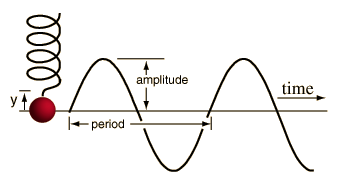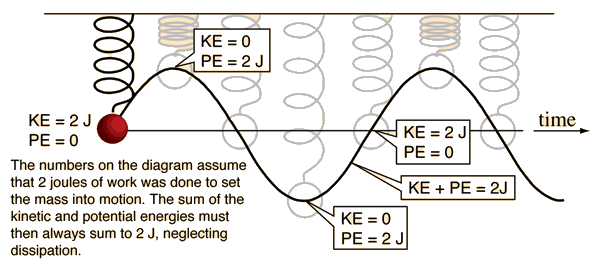# Simple Harmonic Motion

When a mass is acted upon by an elastic force which tends to bring it back to its equilibrium configuration, and when that force is proportional to the distance from equilibrium (e.g., doubles when the distance from equilibrium doubles, a Hooke's Law force), then the object will undergo simple harmonic motion when released.A mass on a spring is the standard example of such periodic motion. If the displacement of the mass is plotted as a function of time, it will trace out a pure sine wave.

### Energy of simple harmonic motion

It turns out that the motion of the medium in a traveling wave is also simple harmonic motion as the wave passes a given point in the medium.

 Mathematical description
Index

Periodic motion concepts

 HyperPhysics***** Sound R Nave
Go Back

# Elasticity and Musical Sources

The precision of return to original configuration after any distortion is a measure of the elasticity of the objects involved. A high degree of elasticity is required of musical sound sources such as violin strings since they are expected to vibrate many thousands of times without stretching enough to be noticeably out of tune. If a string stretches enough to drop its tension by one half of one percent and goes flat from A(440 Hz) to 439 Hz, the acuteness of human pitch perception is such that essentially everybody can hear the difference.

Elasticity can be said to be a precondition for all periodic motion, although the nature of that elasticity may be viewed as a little different from the elasticity of a spring or rubber band, for example. The air supports periodic motion in the form of traveling waves because it has an equilibrium pressure and a restoring force from atmospheric pressure which brings it back to that equilibrium pressure after it has been disturbed.

Index

Periodic motion concepts

 HyperPhysics***** Sound R Nave
Go Back

# Energy of an Oscillator

A mass on a spring transforms energy back and forth between kinetic and potential energy. If there were no dissipation, conservation of energy would dictate that the motion would continue forever. For any real vibrating object, the implication of the conservation of energy principle is that the vibrator will continue the transformation from kinetic to potential energy until all the energy is transferred into some other form. To set the object into motion, a net external force must do work on the mass to initially stretch the spring, that amount of work being 2 joules in the example below.Energy of mass on spring
Index

Periodic motion concepts

 HyperPhysics***** Sound R Nave
Go Back